Smartick is an advanced online program that teaches kids math and coding in only 15 min. a day

Mar30

# Learn How to Add Polynomials with Examples

What are polynomials? Before we start adding them, we need to know what they are and what properties they have. It’s important to understand what factors and degrees are, and how to order the terms.

If you’re not sure about these concepts, you can read these previous posts about the properties of monomials and properties of polynomials.

You can do the addition in two different ways: horizontally and vertically. We’re going to go through both methods, and you can choose the method you prefer afterward.

To do the operations horizontally, first we have to write the polynomials on the same line; the polynomial we are going to add to, followed by the one we are going to add or subtract. Then, we group similar terms together.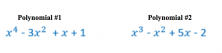Example: We’re going to do this addition. Let’s start by writing out each polynomial inside brackets, with the addition sign between them.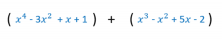Find similar terms between both polynomials. We can’t add two terms with different degrees, we can only group the ones that are similar and add later.

The similar terms are identified using colored circles in the following picture.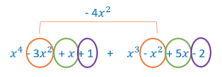Just as we did with the term with the degree of 2, we have to add the terms with a degree of 1 and the terms with a degree of 0.

The result of the addition is:To add vertically, we need to order the polynomials before we start. If the two polynomials don’t contain the same number of terms, it’s best to leave a gap in place of the missing ones. We write the second polynomial underneath the first so that each term coincides with the one above. Now we can add each column.

Example:

We’re going to add the same two polynomials from the previous example vertically.Look at the first polynomial. We have to write it out in order and see if it has the same terms as the second. In this case, there is no term with a degree of 3, so we should leave a gap or write a zero in its place.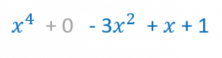Now we write the second polynomial underneath the first so that the similar terms are in the same columns.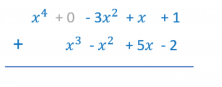Now we just have to add each column, or in other words, add the similar terms.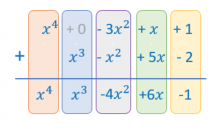You’ve finished learning how to add polynomials using two different methods: horizontal and vertical. Which one did you think was easier?